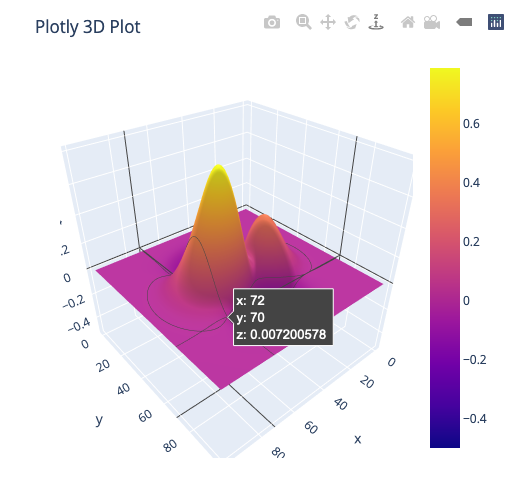# I can't get 3d view

I tried to try panel 3d code which is in panel document with bokeh serve.
But I got:
" bokeh] Failed to load Bokeh session 9RcjbdRciYMohUeOFWBoe3uPxdTDbfP5Kai4IG1Rfsf6: Error: Model ‘panel.models.plotly.PlotlyPlot’ does not exist. This could be due to a widget or a custom model not being registered before first usage. "

``````import panel as pn
pn.extension('plotly')

import numpy as np
import plotly.graph_objs as go

xx = np.linspace(-3.5, 3.5, 100)
yy = np.linspace(-3.5, 3.5, 100)
x, y = np.meshgrid(xx, yy)
z = np.exp(-(x-1)**2-y**2)-(x**3+y**4-x/5)*np.exp(-(x**2+y**2))

surface = go.Surface(z=z)
layout = go.Layout(
title='Plotly 3D Plot',
autosize=False,
width=500,
height=500,
margin=dict(t=50, b=50, r=50, l=50)
)
fig = dict(data=[surface], layout=layout)

plotly_pane = pn.pane.Plotly(fig)
plotly_pane.servable()
``````

I reinstall related libraries. But I couldn’t get the 3d view.
here are the versions
panel 0.9.5
plotly-4.8.1-py_0
holoviews 1.13.2
bokeh 2.0.1

regards

Hi @Ahmet

Try updating bokeh to 2.0.2. There is a know bug with Bokeh 2.0.1 . The bug is discussed here https://github.com/holoviz/panel/issues/1205.

If it works for you please mark this as the solution.

Thanks.

1 Like

Hi
Sorry to say this.

The code you posted works for me (I show it below, where I just changed `servable` with `show`) with Bokeh 2.0.2 so I think @Marc is correct. Have you tried updating also Plotly?

I have these versions in my environment:
bokeh 2.0.2
panel 0.9.5
python 3.7.7
plotly 4.8.2

``````import panel as pn
pn.extension('plotly')

import numpy as np
import plotly.graph_objs as go

xx = np.linspace(-3.5, 3.5, 100)
yy = np.linspace(-3.5, 3.5, 100)
x, y = np.meshgrid(xx, yy)
z = np.exp(-(x-1)**2-y**2)-(x**3+y**4-x/5)*np.exp(-(x**2+y**2))

surface = go.Surface(z=z)
layout = go.Layout(
title='Plotly 3D Plot',
autosize=False,
width=500,
height=500,
margin=dict(t=50, b=50, r=50, l=50)
)
fig = dict(data=[surface], layout=layout)

plotly_pane = pn.pane.Plotly(fig)
plotly_pane.show()
``````1 Like

Hi,
It worked with plotly/dash.
thank you